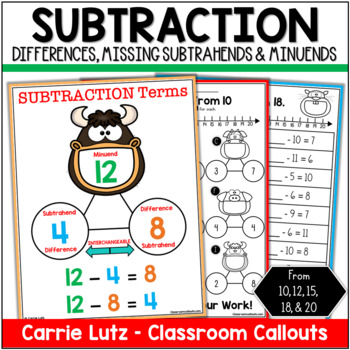Subtraction Strategies: Finding Differences, Missing Minuends & Subtrahends1st - 2nd, Homeschool
Subjects
Standards
Resource Type
Formats Included
• PDF
Pages
40 pages

Description

Teaching missing numbers in subtraction sentences requires higher order thinking practice. This resource has over 30 no-prep pages that can help your student learn how to solve for the difference, missing subtrahend, and missing minuend. If you aren't sure what these terms mean, that's ok! There are helpful posters included that will help your kids grasp the terms and actually use them appropriately!

Why use these terms: The math standards are clear for both the Common Core and the TEKS.

Common Core - Determine the unknown whole number in an addition or subtraction equation relating three whole numbers. For example, determine the unknown number that makes the equation true in each of the equations 8 + ? = 11, 5 = _ - 3, 6 + 6 = _.

TEKS - Use objects and pictorial models to solve word problems involving joining, separating, and comparing sets within 20 and unknowns as any one of the terms in the problem such as 2 + 4 = [ ]; 3 + [ ] = 7; and 5 = [ ] - 3.

Here's what's included:

• Subtraction Terms Poster showing a subtraction sentence in a number bond. The numbers are labeled with Difference, Minuend and Subtrahend.
• Blank Number Bond Poster with a number line and labels (difference, minuend, subtrahend).
• Directions for use.
• How to solve problems using a number line (included on each worksheet).
• Worksheet Differentiation - Differences, Minuends and Subtrahend from 10, 12, 15, 18, 20
• 2 Different Worksheets Styles - Basic Fact and Number Bonds
• 30 Worksheet in all

Let’s Connect!
If you would like to get updates on NEW and CURRENT resources...

Total Pages
40 pages
N/A
Teaching Duration
N/A
Report this Resource to TpT
Reported resources will be reviewed by our team. Report this resource to let us know if this resource violates TpT’s content guidelines.

Standards

to see state-specific standards (only available in the US).
Determine the unknown whole number in an addition or subtraction equation relating three whole numbers. For example, determine the unknown number that makes the equation true in each of the equations 8 + ? = 11, 5 = ▯ - 3, 6 + 6 = ▯.